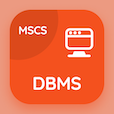Engineering Online Courses

Engineering Mathematics Quizzes

Engineering Mathematics Quiz PDF - Complete

# Basic Concepts Quiz Questions Online p. 43

Learn Basic Concepts quiz questions and answers, basic concepts MCQ with answers PDF 43 to learn Engineering Mathematics online course. First Order Ordinary Differential Equations trivia questions, Basic Concepts Multiple Choice Questions (MCQ) for online college degrees. "Basic Concepts Quiz" PDF Book: logarithm, number types, basic concepts test prep for online engineering associate's degree.

"A number of coupled differential equations form a" MCQ PDF: solution of equations, couple of equations, systems of equations, and differential equations for questions to ask during an interview. Study first order ordinary differential equations questions and answers to improve problem solving skills for online engineering associate's degree.

## Quiz on Basic Concepts MCQs

MCQ: A number of coupled differential equations form a

couple of equations
solution of equations
systems of equations
differential equations

MCQ: If in equation p/q, q=1 then it is

irrational equation
rational equation
binary equation
negative equation

MCQ: Briggsian logarithm has base equals to

2
3
10
12

MCQ: A set of real numbers with the property that any number that lies between two numbers in the set is also included in the set is known as

infinite interval
real interval
closed interval
open interval

MCQ: Any number that can be expressed as the quotient or fraction p/q of two integers, a numerator p and a non-zero denominator q is

irrational numbers
rational numbers
binary numbers
negative numbers

### More Quizzes from Engineering Mathematics Course